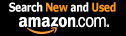CTK Exchange Front Page Movie shortcuts Personal info Awards Reciprocal links Terms of use Privacy Policy Cut The Knot! MSET99 Talk Games & Puzzles Arithmetic/Algebra Geometry Probability Eye Opener Analog Gadgets Inventor's Paradox Did you know?... Proofs Math as Language Things Impossible My Logo Math Poll Other Math sit's Guest book News sit's Recommend this site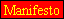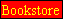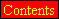|Store|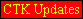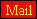CTK Exchange

 Subject: "Puzzle: TI-3" Previous Topic | Next Topic
 ConferencesThe CTK ExchangeMiddle schoolTopic #13Printer-friendly copyEmail this topic to a friend Reading Topic #13
Prashant Poddar
Charter Member
Oct-22-00, 02:15 PM (EST)"Puzzle: TI-3"

 Suppose we have a calculator that is a TI-3, which means that we can only add, subtract and find reciprocals (1/x). Is there still a way for this calculator to multiply numbers? Please explain.

Subject     Author     Message Date     IDRE: Puzzle: TI-3 alexb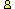Oct-23-00 1RE: Puzzle: TI-3 sfwc Feb-08-04 2RE: Puzzle: TI-3 alexbFeb-08-04 3RE: Puzzle: TI-3 sfwc Feb-08-04 4RE: Puzzle: TI-3 alexbFeb-08-04 5RE: Puzzle: TI-3 sfwc Feb-09-04 6RE: Puzzle: TI-3 D.V.karthickFeb-21-04 7RE: Puzzle: TI-3 alexbFeb-21-04 8RE: Puzzle: TI-3 sfwc Feb-22-04 9RE: Puzzle: TI-3 alexbJul-15-05 10

 Conferences | Forums | Topics | Previous Topic | Next Topic
alexbCharter Member
1580 posts
Oct-23-00, 01:08 AM (EST)1. "RE: Puzzle: TI-3"
In response to message #0

 As I am unfamiliar with TI-3, does it have memory? Also, what kind of numbers do you have in mind? Integers?

sfwc
Member since Jun-19-03
Feb-08-04, 02:04 PM (EST)2. "RE: Puzzle: TI-3"
In response to message #0

 My goodness.. has nobody answered this? It's a pleasant puzzle, and well worth trying.Thankyousfwc<>alexbCharter Member
1580 posts
Feb-08-04, 02:10 PM (EST)3. "RE: Puzzle: TI-3"
In response to message #2

 >My goodness.. has nobody answered this? It's a pleasant >puzzle, and well worth trying. >PS: As far as I know, there is no such calculator actually >manufactured. But there's a Broken Calculator page:https://www.cut-the-knot.org/arithmetic/Calculator.shtml>It's just there to set up the puzzle: >>Can you represent multiplication as a composition of >additions, subtractions and reciprocations? xy = (x + y)/(1/x + 1/y),except that it's unclear whether the reciprocal could indeed be always represented exactly in a TI.sfwc
Member since Jun-19-03
Feb-08-04, 03:10 PM (EST)4. "RE: Puzzle: TI-3"
In response to message #3

 >xy = (x + y)/(1/x + 1/y), That uses division, not reciprocation. More precisely, what you have said is xy = (x + y)*recip(recip(x) + recip(y)), so you are using a hidden multiplication. If you're going to do that, you may as well say xy = x/(1/y). The actual solution is a tad more subtle. As far as I know, the TI-3 is intended to have memory, but I'll take a little time this evening to try to work out if you can do it without memory. Thankyousfwc<><alexbCharter Member
1580 posts
Feb-08-04, 03:54 PM (EST)5. "RE: Puzzle: TI-3"
In response to message #4

 >>xy = (x + y)/(1/x + 1/y), >That uses division, not reciprocation. More precisely, what >you have said is xy = (x + y)*recip(recip(x) + recip(y)), so >you are using a hidden multiplication. If you're going to do >that, you may as well say xy = x/(1/y). The actual solution >is a tad more subtle. As far as I know, the TI-3 is intended >to have memory, but I'll take a little time this evening to >try to work out if you can do it without memory. >Aha, now I see. With (a lot of) memory it's still more or less straightforward. Perhaps, for a simple calculator with only three operations you may want to limit the number of memory registers, or seek a minimumal number. Would be an achievement even then.sfwc
Member since Jun-19-03
Feb-09-04, 10:32 AM (EST)6. "RE: Puzzle: TI-3"
In response to message #5

 Okay, I model the calculator as follows: say it has a stack of size n (this is the memory restriction), and can do the following operations:a: Put the number a onto the stack, or return ERROR if the stack is full. This simulates the user entering the number a, which (s)he presumably knows.b: Put the number b onto the stack, or return ERROR if the stack is full.1: Put the number 1 onto the stack, or return ERROR if the stack is full.+: Take the top 2 numbers off the stack, or return ERROR if the stack only has 1 number on it. Put their sum onto the stack.-: Take the top number off the stack, or return ERROR if the stack is empty. Put minus that number onto the stack.R(k) Where k is a natural number: Take the top number off the stack, or return ERROR if the stack is empty. Put the reciprocal of that number onto the stack, or return ERROR if that number is 0. The number k is just a reference for the user of the calculator. The stack is initally empty. The aim is to come up with a string which will finish with ab as the only number on the stack, for as small a value of n as possible. Of course, if you get ERROR from R(k), you may start again, with a new empty stack, using the new information you have gained. I have refenced what you do if you get ERROR when calculating R(k) after the heading R(k). Here is a solution for n = 3:ab+1+1+R(1)-ab+R(2)+R(3)a1+1+R(4)aR(5)-+R(6)+b1+1+R(7)bR(8)-+R(9)+R(1): a1+R(10)aR(11)-+R(12)a+-R(2): a1+R(13)aR(14)-+R(15)-a+R(3): Will never occur.R(4): bb+-R(5): 1-1+R(6): Will never occur.R(7): aa+-R(8): 1-1+R(9): Will never occur.R(10): 1R(11): 1-1+R(12): Will never occur.R(13): 1-R(14): 1-1+R(15): Will never occur.Although that looks a little complex, what it is doing is simple enough. I guess I must have made a mistake somewhere in there, so feel free to correct it.There is no solution for n = 2. I leave this as a challenge for the moment, but will post a proof if you want me to.Thankyousfwc<><

D.V.karthickguest
Feb-21-04, 01:24 AM (EST)

7. "RE: Puzzle: TI-3"
In response to message #0

 hello! my dear guy there is a great posibility for the multiplication because let us take the number be X then now reverse the number it is 1/X now when you add the reverse number to the same number or just a new number say Y then for taking the L.C.M. and going on with the problem then it would be muliplied with that number now the result would be (1+X.Y)/X nbow subtract the 1/X from it then it'shall become the same Y.sorry I AM NOT ABLE TO FIND IT!!!!alexbCharter Member
1580 posts
Feb-21-04, 01:47 AM (EST)8. "RE: Puzzle: TI-3"
In response to message #7

 Takes a little perseverance. Let the two numbers be a and b. Try the following steps (overlooking any exceptions):1/(x-1) + (y-1) = <1 + (x-1)(y-1)>/(x-1) (reciprocal and sum)(x-1)/<1 + (x-1)(y-1)> (reciprocal)1/(y-1) + (x-1) = <1 + (y-1)(x-1)>/(y-1) (reciprocal and sum)(y-1)/<1 + (y-1)(x-1)> (reciprocal)(x+y-2)/<1 + (x-1)(y-1)> = (x+y-2)/(2-x-y+xy) (sum)(2-x-y+xy)/(x+y-2) = -1 + xy/(x+y-2) (reciprocal)xy/(x+y-2) (add 1)(x+y-2)/xy (reciprocal)1/y + 1/x - 2/xy 1/x + 1/y (2 reciprocals and sum)(1/x + 1/y) - (1/y + 1/x - 2/xy) (subtract)2/xyxy/2 (reciprocal)xy/2 + xy/2 = xy (sum)sfwc
Member since Jun-19-03
Feb-22-04, 07:06 AM (EST)9. "RE: Puzzle: TI-3"
In response to message #8

 As I said in my previous post, we may do the following:1/x - 1/(x+2) = 2/x(x+2)1/y - 1/(y+2) = 2/y(y+2)1/(x+y) - 1/(x+y+2) = 2/(x+y)(x+y+2)(x+y)(x+y+2)/2 - x(x+2)/2 - y(y+2)/2 = xy.This seems a little simpler (and it only needs a stack size of 3, which was why I posted it).Thankyousfwc<><alexbCharter Member
1580 posts
Jul-15-05, 07:21 PM (EST)10. "RE: Puzzle: TI-3"
In response to message #8

 In Five Hundred Mathematical Challenges by E. Barbeau et al this is problem #346. Comes with two solutions, too. I like the second one:If u is not 1, u2 = u - 1/(1/u + 1/(1-u)).u/2 = 1/(1/u + 1/u).uv = ((u+v)/2)2 - ((u-v)/2)2.

 Conferences | Forums | Topics | Previous Topic | Next Topic
 Select another forum or conference Lobby The CTK Exchange (Conference)   |--Early math (Public)   |--Middle school (Public)   |--High school (Public)   |--College math (Public)   |--This and that (Public)   |--Guest book (Public) Educational Press (Conference)   |--No Child Left Behind (Public)   |--Math Wars (Public)   |--Mathematics and general education (Public)You may be curious to have a look at the old CTK Exchange archive.